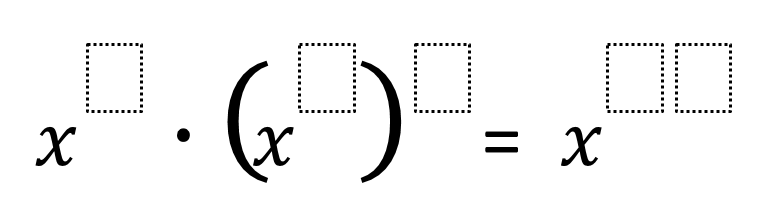# Exponential Powers

Directions: Using the digits 0 to 9 at most one time each, place a digit in each box to create a result with the greatest exponent.### Hint

Which part of the equation will be the most important to start with when trying to create the largest exponent?

X^4 * (x^9)^8 = x^76

Source: Kjersti Oliver

## Interior and Exterior Angles of Triangles

Directions: In triangle ABC, angle ABC is obtuse. Using the digits 1 to 9 at …

### One comment

1.This is an excellent resource – thank you !